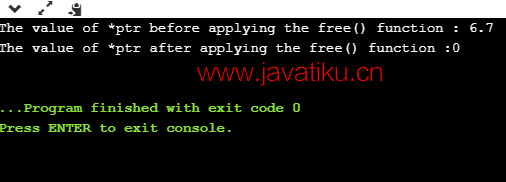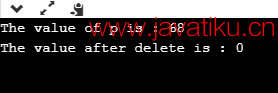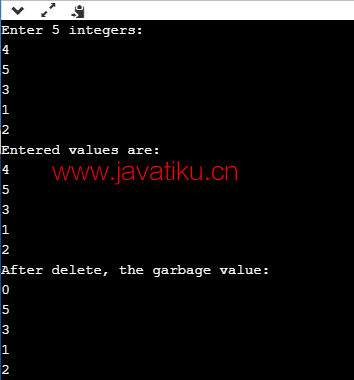# C++教程-C++free() vs delete ()## C++ free() vs delete()

### free() 函数

#### free() 函数的语法

``free(ptr); ``

#### free() 函数参数

• 如果指针是空指针，则 free() 函数不会执行任何操作。
• 如果指针是使用 malloc、calloc 或 realloc 函数分配的，但没有指向任何内存块，则该函数会导致未定义行为。 free() 返回值 free() 函数不返回任何值。它的主要作用是释放内存。

``````#include <iostream>
#include <cstdlib>
using namespace std;

int main()
{
int *ptr;
ptr = (int*) malloc(5*sizeof(int));
cout << "Enter 5 integers" << endl;

for (int i=0; i<5; i++)
{
// *(ptr+i) can be replaced by ptr[i]
cin >>ptr[i];
}
cout << endl << "User entered values" << endl;

for (int i=0; i<5; i++)
{
cout <<*(ptr+i)  << " ";
}
free(ptr);

/* prints a garbage value after ptr is freed */
cout << "Garbage Value" << endl;

for (int i=0; i<5; i++)
{
cout << *(ptr+i)<< " ";
}
return 0;
}````````````#include <iostream>
#include <cstdlib>
using namespace std;
int main()
{
float *ptr; // 浮点型指针声明
ptr = (float*)calloc(1,sizeof(float));
*ptr=6.7;
std::cout << "The value of *ptr before applying the free() function : " <<*ptr<< std::endl;
free(ptr);
std::cout << "The value of *ptr after applying the free() function :" <<*ptr<< std::endl;
return 0;
}````````````#include <iostream>
#include <cstdlib>
using namespace std;
int main()
{
int *ptr1=NULL;
int *ptr2;
int x=9;
ptr2=&x;
if(ptr1)
{
std::cout << "Pointer is not Null" << std::endl;
}
else
{
cout<<"Ponter is NULL";
}
free(ptr1);
//free(ptr2); // 如果执行这条语句，会出现运行时错误。
return 0;
}``````### Delete 运算符

Delete 运算符是在 C++ 编程语言中使用的运算符，用于动态释放内存。它主要用于删除使用 new 运算符分配的指针或空指针。

#### 语法

``delete pointer_name ``

``delete p; ``

``delete [] p; ``

• 它用于删除使用 new 关键字分配的数组或非数组对象。
• 为了删除数组或非数组对象，我们分别使用 delete[] 和 delete 运算符。
• new 关键字在堆中分配内存，因此可以说 delete 运算符总是从堆中释放内存。 它不会销毁指针，而是销毁指针所指向的值或内存块。

``````#include <iostream>
#include <cstdlib>
using namespace std;

int main()
{
int *ptr;
ptr = new int;
*ptr=68;
std::cout << "The value of p is : " <<*ptr<< std::endl;
delete ptr;
std::cout <<"The value after delete is : "  <<*ptr<< std::endl;
return 0;
}````````````#include <iostream>
using namespace std;
int main()
{
int *ptr=new int;  // 使用 new 运算符分配内存。
std::cout << "输入 5 个整数:" << std::endl;
for(int i=1;i<=5;i++)
{
cin>>ptr[i];
}
std::cout << "输入的值为:" << std::endl;
for(int i=1;i<=5;i++)
{
cout<<*(ptr+i)<<endl;
}
delete[] ptr; // 删除指针所指向的内存块。
std::cout << "delete 后的垃圾值:" << std::endl;
for(int i=1;i<=5;i++)
{
cout<<*(ptr+i)<<endl;
}
return 0;
}``````### delete 和 free() 的区别

delete 和 free() 在 C++ 中的区别如下：

• delete 是一个动态释放内存的运算符，而 free() 是一个在运行时销毁内存的函数。
• delete 运算符用于删除指针，该指针可以是使用 new 运算符分配的指针或空指针，而 free() 函数用于删除使用 malloc()、calloc() 或 realloc() 函数分配的指针或空指针。
• 当 delete 运算符销毁已分配的内存时，它会调用 C++ 类的析构函数，而 free() 函数不会调用析构函数，它只会释放堆中的内存。
• delete() 运算符比 free() 函数快。